# Disjoint

How many elements have union and intersection of two disjoint sets when the first have 1 and secodn 8 elements.

union:  9
intersect:  0

### Step-by-step explanation:

$n\left(A\cup B\right)=n\left(A\right)+n\left(B\right)=1+8=9$
$n\left(A\cap B\right)=0$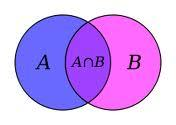Did you find an error or inaccuracy? Feel free to write us. Thank you!## Related math problems and questions:

• Subtracting sets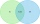For two sets K, L is true: K has 30 elements, L has 27 elements and the set L - K has 22 elements. How many elements does the set K - L have?
• Two sets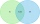Suppose Set B contains 69 elements and the total number elements in either Set A or Set B is 124. If the Sets A and B have 29 elements in common, how many elements are contained in set A?
• Operations with sets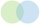The set B - A has twice as fewer elements as the set A - B and four times fewer elements than the set A ∩ B. How many times more elements does the set A have than the set B?
• Intersect of setsRefer to the sets below: U = {1,2,3,. .. ,10} A = {1,2,3,4,5} B = {2,4,6,7,8} C = {4,5,6,8,10} A ∩ B ∩ C = ___
• Class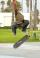The class has 18 students. Everyone knows inline skating or skateboarding. Inline skating can ride 11 students on a skateboard 10. How many ride on inline skates and on skateboard?
• Language coursesOf the company's 60 employees, 28 attend an English course, 17 take a German course, and 20 do not attend any of these courses. How many employees attend both courses?
• News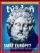From 28 students buy magazine 10 The economist students, 10 Pravda students and 18 students don't buy any of these magazines. How many students buy both magazines?
• Brothers and sistersThere are 35 children in the class, 23 of them have a brother, and 27 of them have a sister. How many children have both a brother and a sister when there are 5 children in the class who have no brother or sister?
• Group of childrenThere is a group of children. There is a boy named Adam in each of the three children subgroup and a girl named Beata in each quartet (four-member subgroup). How many children can be in such a group and what are their names in that case?
• Camp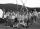In a class are 26 children. During the holidays 16 children were in the camps and 14 children on holiday with their parents. Determine the minimum and maximum number of children that may have been in the camp and on holiday with their parents at the same
• 80 students80 students were asked what type of test they preferred. 50 students said they liked multiple choice and 42 liked true or false. If 36 liked both multiple choice and true or false types, how many students preferred multiple choice only?
• SegmentsLine segments 62 cm and 2.2 dm long we divide into equal parts which lengths in centimeters is expressed integer. How many ways can we divide?
• There 11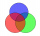There are 50 pupil in the class. Out of of this number,1/10 speak French only and 4/5 of the 10 remainder speak both French and English . If the rest speak English only find the number of students who speak only English?
• CombinationsIf the number of elements increase by 3, it increases the number of combinations of the second class of these elements 5 times. How many are the elements?
• Marriage sttusIn our city, there are 3/5 of the women married to 2/3 of the men. Find what part of the population is free.
• Venn diagramUniversity students chose a foreign language for the 1st year. Of the 120 enrolled students, 75 chose English, 65 German, and 40 both English and German. Using the Venn diagram, determine: - how many of the enrolled students chose English only - how many
• Elements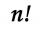If the number of elements is decreased by two the number of permutations is decreased 30 times. How many elements are?